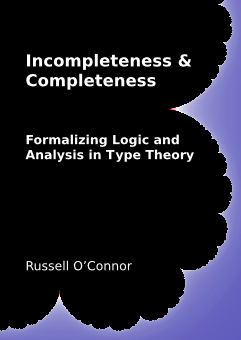# PhD Thesis

## Incompleteness & Completeness:Formalizing Logic and Analysis in Type Theory### Summary

This thesis covers two developments in constructive type theory: a proof of Gödel's first incompleteness theorem and a development of exact real arithmetic. I work in constructive type theory because this system has the unique ability to freely mix dependently typed programming and natural deduction style proofs. Through the Curry-Howard isomorphism, these two tasks are accomplished with exactly the same language. Both the programming aspect and the proof aspect of this language support each other. Proofs are used to certify the correctness of functions, and correct functions can be evaluated in proofs to solve problems.

Chapter 1 and Chapter 2 introduce the topic of my thesis and provides a brief introduction to the kind of type theory I will be using. Both developments have been formalized with the Coq proof assistant which implements constructive type theory. I also show how classical logic can be seen as a fragment of constructive logic. In this way, classical reasoning is also supported in constructive type theory.

In Part I, I describe my development of Gödel's first incompleteness theorem. It begins with Chapter 3 which provides a brief introduction and a description of the incompleteness theorem.

The description of my formal proof begins in Chapter 4 where I describe my data structure for first order formulas and my data structure for proofs in first order logic. I also define two axiom systems: Peano arithmetic and a very weak arithmetic system called NN. This internal logic is classical, whereas Coq's logic is constructive. I chose a traditional definition of substitution in formulas where bound variables are renamed in order to avoid variable capture. This choice caused many difficulties throughout the proof.

Chapter 5 discusses how I encode various structures as natural numbers and how I show that the functions operating on these structures can be represented by arithmetic formulas. Rather than using the prime decomposition theorem to encode strings, as Gödel originally did, I recursively used the Cantor pairing function to encode the abstract syntax trees of formulas and proofs. In Section 5.2, I defined a language for primitive recursive functions. I wrote primitive recursive programs for all the needed functions on formulas and proofs. I proved that these primitive recursive programs were correct representations of the original Coq functions. In Section 5.4, I describe how I proved that all primitive recursive functions can be represented by arithmetic formulas.

Chapter 6 gives my formal statement of the incompleteness theorem. I proved that system NN is essentially incomplete. In particular, Peano arithmetic is incomplete because I also proved that Peano arithmetic is consistent by showing that Coq's natural numbers form a model of Peano arithmetic. Finally, Chapter 7 gives a few concluding remarks about this proof and describes what more will be needed to prove the second incompleteness theorem.

In Part II, I describe my implementation of exact real arithmetic by first creating a general implementation of complete metric spaces. This part begins with Chapter 8 which provides a brief introduction and some background on Bishop and Bridges's approach to real numbers.

Chapter 9 introduces my formal definition of a metric space and various classifications of metric spaces having additional properties. Uniformly continuous functions between metric spaces are defined. They play a central role in this work.

Chapter 10 defines the completion operation which creates a complete metric space from an arbitrary metric space. I show that this completion operation has the required functions to make it into a monad. I define operations to lift uniformly continuous functions over metric spaces to uniformly continuous functions over the completion of those metric spaces.

Chapter 11 defines the real numbers as the completion of the rational numbers. The field operations for the real numbers are defined by lifting the operations from the rational numbers. Other elementary functions are defined first on the rational numbers by computing the limit of power series. Then they too are lifted so they can operate on all real numbers. Section 11.5 details ways that some common operations can be defined more efficiently. All my functions can be executed inside Coq and are efficient enough that computing approximations is practical. I wrote a tactic that uses computation to solve inequalities between closed real number expressions.

The purpose of my work on complete metric spaces was to define the real numbers. However, my implementation of complete metric spaces is general. To illustrate this, Chapter 12 defines the metric space of integrable functions as the completion of formal step functions with the L1 metric. Uniformly continuous functions can be mapped to integrable functions on [0,1] and integrated. Section 12.1.8 shows how a Stieltjes integral falls out of this work for free.

Chapter 13 illustrates another application of complete metric spaces, this time to compact sets. The metric space of compact sets is defined as the completion of the space of finite sets with the Hausdorff metric. This means that every compact set can be approximated by a finite set. Furthermore, this finite set can be rasterized and displayed inside the Coq system. For example, I show that the graph of any uniformly continuous function over a compact interval is compact. Figure 13.1 shows an approximation to the exponential function drawn in Coq. Section 13.2.2 shows a nice example of how one can mix classical and constructive reasoning. Section 13.6 discusses why I think that constructive logic is a better language for talking about computability than classic computability theory.

Finally, Chapter 14 ends with some conclusions reached from this research.

Russell O’Connor: contact me